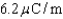Try out our new practice tests completely free!# College Physics Reasoning and Relationships

Physics & Astronomy

## Quiz 17 : Electric Forces and FieldsStudy FlashcardsBookmarkLooking for Introductory Physics Homework Help?Bookmark

## Quiz 17 : Electric Forces and FieldsAbout how many electrons are in 0.3 gram of water (H2O)?
Free
Multiple Choice

AObject A has a charge q on it, object B has a charge q on it, and object C has a charge 2q on it. These charges are arranged, one each, at the vertices of an equilateral triangle. Which charge has the greatest magnitude electric force on it?
Free
Multiple Choice

BTwo identical iron spheres have equal positive charges and the force between them when they are 1 m apart is 4 N. What percentage of the electrons has been removed from each sphere if each sphere has 1 mole (6 1023) of iron atoms? (ke = 8.99 109 Nm2/C2, the atomic number of iron is 26, and the electron charge is 1.6 1019 C)
Free
Multiple Choice

ATwo point charges, separated by 15 cm, have charge values of +2.0 µC and 4.0 µC, respectively. What is the value of the mutual force between them? (ke = 8.99 109 Nm2/C2)
Multiple ChoiceThe constant ke, which appears in Coulomb's law formula, is equivalent dimensionally to which of the following?
Multiple ChoiceTwo identical balls have the same amount of charge, but the charge on ball A is positive and the charge on ball B is negative. The balls are placed on a smooth, level, frictionless table whose top is an insulator. Which of the following is true?
Multiple ChoiceIf the size of the charge value is tripled for one of two point charges maintained at a constant separation, the mutual force between them will be changed by what factor?
Multiple ChoiceIf the distance between two point charges is quadrupled, the mutual force between them will be changed by what factor?
Multiple ChoiceTwo identical spheres each carry a charge of 40.0 µC. The spheres are separated by a distance of 2.00 m. What is the electric force between the spheres? (ke = 8.99 109 N·m2/C2)
Multiple ChoiceA repelling force must occur between two charged objects under which conditions?
Multiple ChoiceDoug rubs a piece of fur on a hard rubber rod, giving the rod a negative charge. What happens?
Multiple ChoiceTwo electrons are separated by one cm. What is the ratio of the electric force to the gravitational force between them? (me = 9.11 1031 kg, ke = 8.99 109 Nm2/C2, G = 6.67 10-11 Nm2/kg2, and e = 1.6 1019 C)
Multiple ChoiceThe charge per unit length on an insulator is. On a 2.0-m length of this material, what is the difference in the number of electrons and the number of protons?
Multiple ChoiceTwo point charges are 4 cm apart. They are moved to a new separation of 8 cm. By what factor does the resulting mutual force between them change?
Multiple ChoiceA 6.00-C charge is placed at the origin and a second charge is placed on the x axis at x = 0.300 m. If the resulting force on the second charge is 6.40 N in the negative x direction, what is the force on the charge at the origin?
Multiple ChoiceA 6.0-mC charge is placed at the origin and a second charge is placed on the x axis at x = 0.30 m. If the resulting force on the second charge is 5.4 N in the positive x direction, what is the value of its charge?
Multiple ChoiceAn electron is sent at high speed toward a gold nucleus (charge +79e). What is the electrical force acting on the electron when it is 3.0 1013 m away from the gold nucleus? (e = 1.6 1019 C, ke = 8.99 109 N·m2/C2)
Multiple Choice